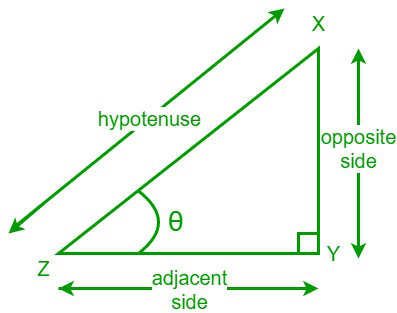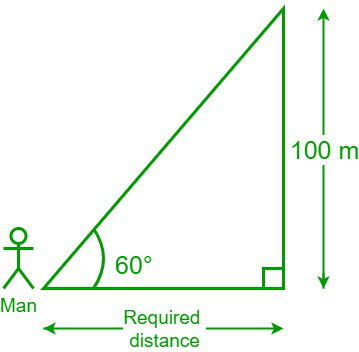Open in App
Not now

# Tangent Formulas

• Last Updated : 02 Nov, 2022

Trigonometry is an important branch of mathematics that deals with the relationship between angles and lengths of the sides of a right-angled triangle. The six trigonometric ratios or functions are sine, cosine, tangent, cosecant, and secant, and a trigonometric ratio is a ratio between the sides of a right-angled triangle. Sine, cosine, and tangent functions are three important trigonometric functions since the other three, i.e., cosecant, secant, and cotangent functions are the reciprocal functions of sine, cosine, and tangent functions, respectively.

• sin θ = Opposite side/Hypotenuse
• cos θ = Adjacent side/Hypotenuse
• tan θ = Opposite side/Adjacent side
• cosec θ = Hypotenuse/Opposite side
• sec θ = Hypotenuse/Adjacent side
• cot θ = Adjacent side/Opposite side

## Tangent Formula

The tangent of an angle in a right-angled triangle is the ratio of the length of the opposite side to the length of the adjacent side to the given angle. We write a tangent function as “tan”. Let us consider a right-angled triangle XYZ and one of its acute angles is “θ”. An opposite side is the side that is opposite to the angle “θ” and the adjacent side is the side that is adjacent to the angle “θ”.Now, the tangent formula for the given angle “θ” is,

tan θ = Opposite side/Adjacent side

## Some Basic Tangent Formulae

The tangent function is positive in the first and third quadrants and negative in the second and fourth quadrants.

tan (2π + θ) = tan θ (1st quadrant)

tan (π – θ) = – tan θ (2nd quadrant)

tan (π + θ) = tan θ (3rd quadrant)

tan (2π – θ) = – tan θ (4th quadrant)

### Tangent Function as a Negative Function

The tangent function is a negative function since the tangent of a negative angle is the negative of a tangent positive angle.

tan (-θ) = – tan θ

### Tangent Function in Terms of Sine and Cosine Function

The tangent function in terms of sine and cosine functions can be written as,

tan θ = sin θ/cos θ

We know that, tan θ = Opposite side/Adjacent side

Now, divide both the numerator and denominator with hypotenuse

tan θ = (Opposite side/Hypotenuse)/(Adjacent side/Hypotenuse)

We know that, sin θ = opposite side/hypotenuse

Hence, tan θ = sin θ/cos θ

### Tangent Function in Terms of the Sine Function

The tangent function in terms of the sine function can be written as,

tan θ = sin θ/(√1 – sin2 θ)

We know that,

tan θ = sin θ/cos θ

From the Pythagorean identities, we have,

sin2 θ + cos2 θ = 1

cos2 θ = 1 – sin2 θ

cos θ = √(1 – sin2 θ)

Hence, tan θ =  sin θ/(√1 – sin2 θ)

### Tangent Function in Terms of the Cosine Function

The tangent function in terms of the cosine function can be written as,

tan θ = (√1 -cos2 θ)/cos θ

We know that,

tan θ = sin θ/cos θ

From the Pythagorean identities, we have,

sin2 θ + cos2 θ = 1

sin2 θ = 1 – cos2 θ

sin θ = √(1 – cos2 θ)

Hence, tan θ =  (√1 – cos2 θ)/cos θ

### Tangent Function in Terms of the Cotangent Function

The tangent function in terms of the cotangent function can be written as,

tan θ = 1/cot θ

or

tan θ = cot (90° – θ) (or) cot (π/2 – θ)

### Tangent Function in Terms of the Cosecant Function

The tangent function in terms of the cosecant function can be written as,

tan θ = 1/√(cosec2 θ – 1)

From the Pythagorean identities, we have,

cosec2 θ – cot2 θ = 1

cot2 θ = cosec2 θ – 1

cot θ = √(cosec2 θ – 1)

We know that,

tan θ = 1/cot θ

Hence, tan θ = 1/√(cosec2 θ – 1)

### Tangent Function in Terms of the Secant Function

The tangent function in terms of the secant function can be written as,

tan θ = √sec2 θ – 1

From the Pythagorean identities, we have,

sec2 θ – tan2 θ = 1

tan θ = sec2 θ – 1

Hence, tan θ = √(sec2 θ – 1)

### Tangent Function in Terms of Double Angle

The tangent function for a double angle is,

tan 2θ = (2 tan θ)/(1 – tan2 θ)

### Tangent Function in Terms of Triple Angle

The tangent function for a triple angle is,

tan 3θ = (3 tan θ – tan3θ) / (1 – 3 tan2θ)

### Tangent Function in Terms of Half-Angle

The tangent function for a half-angle is,

tan (θ/2) = ± √[ (1 – cos θ) / (1 + cos θ) ]

tan (θ/2) = (1 – cos θ) / ( sin θ)

### Tangent Function in Terms of the Addition and Subtraction of Two Angles

The sum and difference formulas for a tangent function are,

tan (A + B) = (tan A + tan B)/(1 – tan A tan B)

tan (A – B) = (tan A – tan B)/(1 + tan A tan B)

## Solved Example on Tangent Formulas

Example 1: Find the value of tan θ if sin θ = 2/5 and θ is the first quadrant angle.

Solution:

Given, sin θ = 2/5

From the Pythagorean identities we have,

sin2 θ + cos2 θ = 1

cos2 θ = 1 – sin2 θ = 1 – (2/5)2

cos2 θ = 1 – (4/5) = 21/25

cos θ = ±√21/5

Since θ is the first quadrant angle, cos θ is positive.

cos θ = √21/5

We know that,

tan θ = sin θ/cos θ

= (2/3)/(√21/5)

Hence, tan θ = 10/3√21

Example 2: Find the value of tan x if sec x = 13/12 and x is the fourth quadrant angle.

Solution:

Given, sec x = 13/12

From the Pythagorean identities, we have,

sec2 x – tan2 x = 1

tan2 x = sec2 x – 1= (13/12)2 – 1

tan2 x = (169/144) – 1= 25/144

tan x = ± 5/12

Since x is the fourth quadrant angle, tan x is negative.

tan x = – 5/12

Hence, tan x = – 5/12

Example 3: If tan X = 2/3 and tan Y = 1/2, then what is the value of tan (X + Y)?

Solution:

Given,

tan X = 2/3 and tan Y = 1/2

We know that,

tan (X + Y) = (tan X + tan Y)/(1 – tan X tan Y)

tan (X + Y) = [(2/3) + (1/2)]/[1 – (2/3)×(1/2)]

= (7/6)/(2/3) = 7/4

Hence, tan (X + Y) = 7/4

Example 4: Calculate the tangent function if the adjacent and opposite sides of a right-angled triangle are 4 cm and 7 cm, respectively.

Solution:

Given,

Opposite side = 7 cm

We know that,

tan θ = Opposite side/Adjacent side

tan θ = 7/4 = 1.75

Hence, tan θ = 1.75

Example 5: A man is looking at a clock tower at a 60° angle to the top of the tower, whose height is 100 m. What is the distance between the man and the foot of the tower?

Solution:

Given,

The height of the tower = 100 m and θ = 60°

Let the distance between the man and the foot of the tower = dWe have,

tan θ = Opposite side/Adjacent side

tan 60° = 100/d

√3 = 100/d [Since, tan  60° = √3]

d = 100/√3

Therefore, the distance between the man and the foot of the tower = 100/√3

Example 6: Find the value of tan θ if sin θ = 7/25 and sec θ = 25/24.

Solution:

Given,

sin θ = 7/25

sec θ = 25/24

We know that,

sec θ = 1/cos θ

25/24 = 1/cos θ cos θ = 24/25

We have,

tan θ = sin θ/cos θ

= (7/25)/(24/25)

= 7/24

Hence, tan θ = 7/24

Example 7: Find the value of tan θ if cosec θ = 5/3, and θ is the first quadrant angle.

Solution:

Given, cosec θ = 5/3

From the Pythagorean identities, we have,

cosec2 θ – cot2 θ = 1

cot2 θ = cosec2 θ – 1

cot θ = (5/3)2 – 1 = (25/9) – 1 = 16/9

cot θ = ±√16/9 = ± 4/3

Since θ is the first quadrant angle, both cotangent and tangent functions are positive.

cot θ = 4/3

We know that,

cot θ = 1/tan θ

4/3 = 1/tan θ

tan θ = 3/4

Hence, tan θ = 3/4

Example 8: Find tan 3θ if sin θ = 3/7 and θ is the first quadrant angle.

Solution :

Given, sin θ = 12/13

From the Pythagorean identities we have,

sin2θ + cos2θ = 1

cos2θ = 1 – sin2θ = 1 – (12/13)2

cos2 θ = 1 – (144/169) = 25/169

cos θ = ±√25/169 = ±5/13

Since θ is the first quadrant angle, cos θ is positive.

cos θ = 5/13

We know that,

tan θ = sin θ/cos θ

= (12/25)/(5/13) = 12/5

Hence, tan θ = 12/5

Now, We know that ,

tan 3θ = (3 tan θ – tan3θ) / (1 – 3 tan2θ)

tan 3θ = 3 × (12/5)

My Personal Notes arrow_drop_up